JEE  >  Test: Scalar Product Of Two Vectors

# Test: Scalar Product Of Two Vectors - JEE

Test Description

## 5 Questions MCQ Test Mathematics (Maths) Class 12 - Test: Scalar Product Of Two Vectors

Test: Scalar Product Of Two Vectors for JEE 2023 is part of Mathematics (Maths) Class 12 preparation. The Test: Scalar Product Of Two Vectors questions and answers have been prepared according to the JEE exam syllabus.The Test: Scalar Product Of Two Vectors MCQs are made for JEE 2023 Exam. Find important definitions, questions, notes, meanings, examples, exercises, MCQs and online tests for Test: Scalar Product Of Two Vectors below.
Solutions of Test: Scalar Product Of Two Vectors questions in English are available as part of our Mathematics (Maths) Class 12 for JEE & Test: Scalar Product Of Two Vectors solutions in Hindi for Mathematics (Maths) Class 12 course. Download more important topics, notes, lectures and mock test series for JEE Exam by signing up for free. Attempt Test: Scalar Product Of Two Vectors | 5 questions in 10 minutes | Mock test for JEE preparation | Free important questions MCQ to study Mathematics (Maths) Class 12 for JEE Exam | Download free PDF with solutions
 1 Crore+ students have signed up on EduRev. Have you?
Test: Scalar Product Of Two Vectors - Question 1

### If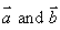are any two vectors, then

Detailed Solution for Test: Scalar Product Of Two Vectors - Question 1

|a+b| ≤ |a| + |b|
Let us take an example : a = 1, b= 2
|1 + 2| ≤ |1| + |2|
|3| ≤ |3|
Hence, proved

Test: Scalar Product Of Two Vectors - Question 2

### The angle between the vectors is: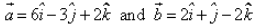is :

Detailed Solution for Test: Scalar Product Of Two Vectors - Question 2

a = 6i - 3j + 2k      b = 2i + j - 2k
a.b = 12 - 3 - 4 = 5
|a| = [(6)2 + (-3)2 + (2)2]1/2
|a| = [36 + 9 + 4]½
|a| = (49)½
|a| = 7
|b| = [(2)2 + (1)2 + (-2)2]½
|b| = [4 + 1 + 4]½
|b| = 3
Cosθ = (a.b)/|a||b|
= 5/(7)(3)
= 5/21
θ = cos-1(5/21)

Test: Scalar Product Of Two Vectors - Question 3

### Ifare two vectors, such that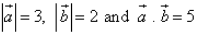, then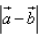= ……​

Detailed Solution for Test: Scalar Product Of Two Vectors - Question 3

|a - b|2 = |a|2 + |b|2 - 2|a||b|
|a - b|2  = (3)2 + (2)2 - 2(5)
|a - b|2  = 9 + 4 - 10
|a - b|2  = 3
|a - b|   = (3)½.

Test: Scalar Product Of Two Vectors - Question 4

The projection of the vector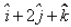on the vector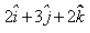is:​

Detailed Solution for Test: Scalar Product Of Two Vectors - Question 4

Projection = (A.B)/|B|
= [(i + 2j + k) . (2i + 3j + 2k)]/[(2)2 + (3)2 + (2)2]½
= (2 + 6 + 2)/[4 + 9 + 4]½
= 10/(17)1/2

Test: Scalar Product Of Two Vectors - Question 5

The angle between two non-zero vectors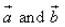is given by

Detailed Solution for Test: Scalar Product Of Two Vectors - Question 5

A sequence is a function whose domain is the set of natural numbers or a subset of the natural numbers. We usually use the symbol an to represent a sequence, where n is a natural number and an is the value of the function on n. A sequence may be finite or infinite.

## Mathematics (Maths) Class 12

206 videos|264 docs|139 tests
Information about Test: Scalar Product Of Two Vectors Page
In this test you can find the Exam questions for Test: Scalar Product Of Two Vectors solved & explained in the simplest way possible. Besides giving Questions and answers for Test: Scalar Product Of Two Vectors, EduRev gives you an ample number of Online tests for practice

## Mathematics (Maths) Class 12

206 videos|264 docs|139 tests